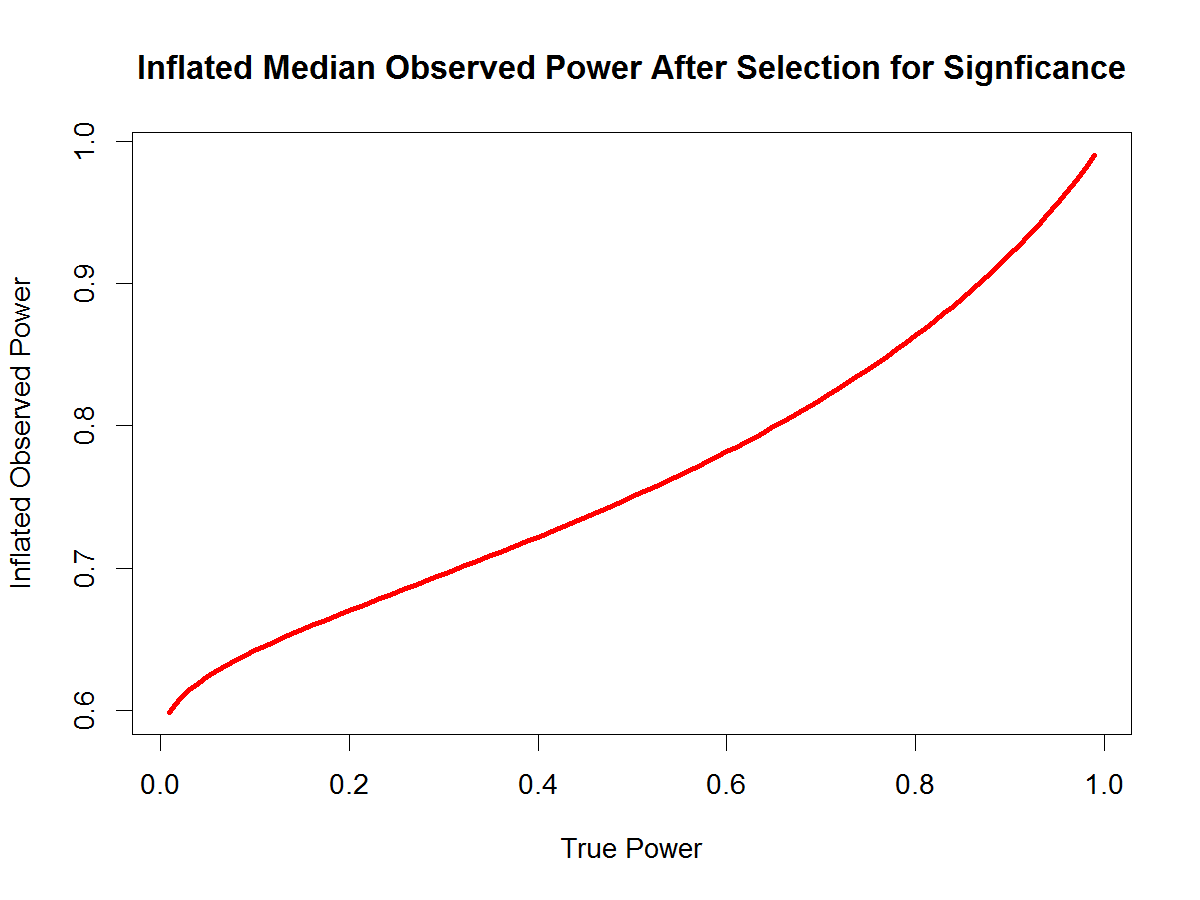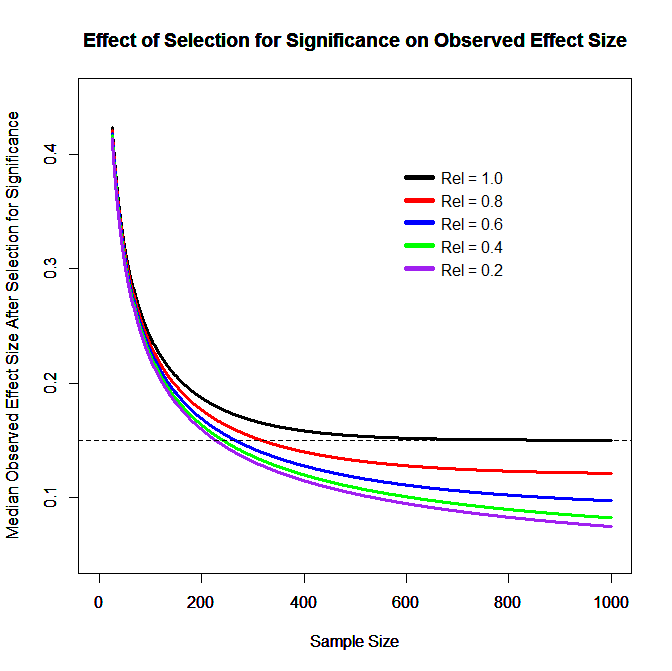# Category Archives: Science Magazine# Random measurement error and the replication crisis: A statistical analysis

This is a draft of a commentary on Loken and Gelman’s Science article “Measurement error and the replication crisis. Comments are welcome.

Random Measurement Error Reduces Power, Replicability, and Observed Effect Sizes After Selection for Significance

Ulrich Schimmack and Rickard Carlsson

In the article “Measurement error and the replication crisis” Loken and Gelman (LG) “caution against the fallacy of assuming that that which does not kill statistical significance makes it stronger” (1). We agree with the overall message that it is a fallacy to interpret observed effect size estimates in small samples as accurate estimates of population effect sizes.  We think it is helpful to recognize the key role of statistical power in significance testing.  If studies have less than 50% power, effect sizes must be inflated to be significant. Thus, all observed effect sizes in these studies are inflated.  Once power is greater than 50%, it is possible to obtain significance with observed effect sizes that underestimate the population effect size. However, even with 80% power, the probability of overestimation is 62.5%. [corrected]. As studies with small samples and small effect sizes often have less than 50% power (2), we can safely assume that observed effect sizes overestimate the population effect size. The best way to make claims about effect sizes in small samples is to avoid interpreting the point estimate and to interpret the 95% confidence interval. It will often show that significant large effect sizes in small samples have wide confidence intervals that also include values close to zero, which shows that any strong claims about effect sizes in small samples are a fallacy (3).

Although we agree with Loken and Gelman’s general message, we believe that their article may have created some confusion about the effect of random measurement error in small samples with small effect sizes when they wrote “In a low-noise setting, the theoretical results of Hausman and others correctly show that measurement error will attenuate coefficient estimates. But we can demonstrate with a simple exercise that the opposite occurs in the presence of high noise and selection on statistical significance” (p. 584).  We both read this sentence as suggesting that under the specified conditions random error may produce even more inflated estimates than perfectly reliable measure. We show that this interpretation of their sentence would be incorrect and that random measurement error always leads to an underestimation of observed effect sizes, even if effect sizes are selected for significance. We demonstrate this fact with a simple equation that shows that true power before selection for significance is monotonically related to observed power after selection for significance. As random measurement error always attenuates population effect sizes, the monotonic relationship implies that observed effect sizes with unreliable measures are also always attenuated.  We provide the formula and R-Code in a Supplement. Here we just give a brief description of the steps that are involved in predicting the effect of measurement error on observed effect sizes after selection for significance.

The effect of random measurement error on population effect sizes is well known. Random measurement error adds variance to the observed measures X and Y, which lowers the observable correlation between two measures. Random error also increases the sampling error. As the non-central t-value is the proportion of these two parameters, it follows that random measurement error always attenuates power. Without selection for significance, median observed effect sizes are unbiased estimates of population effect sizes and median observed power matches true power (4,5). However, with selection for significance, non-significant results with low observed power estimates are excluded and median observed power is inflated. The amount of inflation is proportional to true power. With high power, most results are significant and inflation is small. With low power, most results are non-significant and inflation is large.Schimmack developed a formula that specifies the relationship between true power and median observed power after selection for significance (6). Figure 1 shows that median observed power after selection for significant is a monotonic function of true power.  It is straightforward to transform inflated median observed power into median observed effect sizes.  We applied this approach to Locken and Gelman’s simulation with a true population correlation of r = .15. We changed the range of sample sizes from 50 to 3050 to 25 to 1000 because this range provides a better picture of the effect of small samples on the results. We also increased the range of reliabilities to show that the results hold across a wide range of reliabilities. Figure 2 shows that random error always attenuates observed effect sizes, even after selection for significance in small samples. However, the effect is non-linear and in small samples with small effects, observed effect sizes are nearly identical for different levels of unreliability. The reason is that in studies with low power, most of the observed effect is driven by the noise in the data and it is irrelevant whether the noise is due to measurement error or unexplained reliable variance.In conclusion, we believe that our commentary clarifies how random measurement error contributes to the replication crisis.  Consistent with classic test theory, random measurement error always attenuates population effect sizes. This reduces statistical power to obtain significant results. These non-significant results typically remain unreported. The selective reporting of significant results leads to the publication of inflated effect size estimates. It would be a fallacy to consider these effect size estimates reliable and unbiased estimates of population effect sizes and to expect that an exact replication study would also produce a significant result.  The reason is that replicability is determined by true power and observed power is systematically inflated by selection for significance.  Our commentary also provides researchers with a tool to correct for the inflation by selection for significance. The function in Figure 1 can be used to deflate observed effect sizes. These deflated observed effect sizes provide more realistic estimates of population effect sizes when selection bias is present. The same approach can also be used to correct effect size estimates in meta-analyses (7).

References

1. Loken, E., & Gelman, A. (2017). Measurement error and the replication crisis. Science,

355 (6325), 584-585. [doi: 10.1126/science.aal3618]

2. Cohen, J. (1962). The statistical power of abnormal-social psychological research: A review. Journal of Abnormal and Social Psychology, 65, 145-153, http://dx.doi.org/10.1037/h004518

3. Cohen, J. (1994). The earth is round (p < .05). American Psychologist, 49, 997-1003. http://dx.doi.org/10.1037/0003-066X.49.12.99

4. Schimmack, U. (2012). The ironic effect of significant results on the credibility of multiple-study articles. Psychological Methods, 17(4), 551-566. http://dx.doi.org/10.1037/a0029487

5. Schimmack, U. (2016). A revised introduction to the R-Index. https://replicationindex.com/2016/01/31/a-revised-introduction-to-the-r-index

6. Schimmack, U. (2017). How selection for significance influences observed power. https://replicationindex.com/2017/02/21/how-selection-for-significance-influences-observed-power/

7. van Assen, M.A., van Aert, R.C., Wicherts, J.M. (2015). Meta-analysis using effect size distributions of only statistically significant studies. Psychological Methods, 293-309. doi: 10.1037/met0000025.

################################################################

#### R-CODE ###

################################################################

### sample sizes

N = seq(25,500,5)

### true population correlation

true.pop.r = .15

### reliability

rel = 1-seq(0,.9,.20)

### create matrix of population correlations between measures X and Y.

obs.pop.r = matrix(rep(true.pop.r*rel),length(N),length(rel),byrow=TRUE)

### create a matching matrix of sample sizes

N = matrix(rep(N),length(N),length(rel))

### compute non-central t-values

ncp.t = obs.pop.r / ( (1-obs.pop.r^2)/(sqrt(N – 2)))

### compute true power

true.power = pt(ncp.t,N-2,qt(.975,N-2))

###  Get Inflated Observed Power After Selection for Significance

inf.obs.pow = pnorm(qnorm(true.power/2+(1-true.power),qnorm(true.power,qnorm(.975))),qnorm(.975))

### Transform Into Inflated Observed t-values

inf.obs.t = qt(inf.obs.pow,N-2,qt(.975,N-2))

### Transform inflated observed t-values into inflated observed effect sizes

inf.obs.es = (sqrt(N + 4*inf.obs.t^2 -2) – sqrt(N – 2))/(2*inf.obs.t)

### Set parameters for Figure

x.min = 0

x.max = 500

y.min = 0.10

y.max = 0.45

ylab = “Inflated Observed Effect Size”

title = “Effect of Selection for Significance on Observed Effect Size”

### Create Figure

for (i in 1:length(rel)) {

print(i)

plot(N[,1],inf.obs.es[,i],type=”l”,xlim=c(x.min,x.max),ylim=c(y.min,y.max),col=col[i],xlab=”Sample Size”,ylab=”Median Observed Effect Size After Selection for Significance”,lwd=3,main=title)

segments(x0 = 600,y0 = y.max-.05-i*.02, x1 = 650,col=col[i], lwd=5)

text(730,y.max-.05-i*.02,paste0(“Rel = “,format(rel[i],nsmall=1)))

par(new=TRUE)

}

abline(h = .15,lty=2)

##################### THE END #################################